Welcome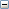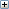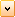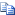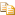IMultiply Method (IMathTransform)

Multiplies two matrices together.

# .NET Syntax

Visual Basic (Declaration)
```Function IMultiply( _
ByVal TransformObjIn As MathTransform _
) As MathTransform```
Visual Basic (Usage)
```Dim instance As IMathTransform
Dim TransformObjIn As MathTransform
Dim value As MathTransform

value = instance.IMultiply(TransformObjIn)```
C#
```MathTransform IMultiply(
MathTransform TransformObjIn
)```
C++/CLI
```MathTransform^ IMultiply(
&   MathTransform^ TransformObjIn
) ```

#### Parameters

TransformObjIn

Math transform by which to multiply the calling math transform

#### Return Value

Newly created math transform object or null if the operation fails

# Remarks

The resulting transform is the result of transforming math transform with respect to the transformObjIn coordinate frame.

# Availability

SOLIDWORKS 2001 FCS, Revision Number 9.0

Provide feedback on this topic

* Required

 *Email: Subject: Feedback on Help Topics Page: IMultiply Method (IMathTransform) *Comment: * I acknowledge I have read and I hereby accept the privacy policy under which my Personal Data will be used by Dassault Systèmes

Print Topic

Select the scope of content to print:

x

We have detected you are using a browser version older than Internet Explorer 7. For optimized display, we suggest upgrading your browser to Internet Explorer 7 or newer.

Never show this message again
x

Web Help Content Version: API Help (English only) 2018 SP05

To disable Web help from within SOLIDWORKS and use local help instead, click Help > Use SOLIDWORKS Web Help.

To report problems encountered with the Web help interface and search, contact your local support representative. To provide feedback on individual help topics, use the “Feedback on this topic” link on the individual topic page.